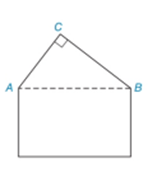Chapter 2.4, Problem 38E### Elementary Geometry for College St...

6th Edition
Daniel C. Alexander + 1 other
ISBN: 9781285195698

#### Solutions

Chapter
Section### Elementary Geometry for College St...

6th Edition
Daniel C. Alexander + 1 other
ISBN: 9781285195698
Textbook Problem
1 views

# The roofline of a house shows the shape of a right triangle A B C with m ∠ C = 90 ° . If the measure of ∠ C A B is 24 ° larger than the measure of ∠ C B A , then how large is each angle?To determine

To find:

The value of mCAB and mCBA.

Explanation

Given:

mC=90° and CAB=24°+CBA

Figure (1)

Approach:

The sum of interior angles of a triangle is equal to 180°.

Calculation:

mCAB+mCBA+mC=180°

Substitute 24°+CBA for CAB, and 90° for mC in equation (1).

### Still sussing out bartleby?

Check out a sample textbook solution.

See a sample solution

#### The Solution to Your Study Problems

Bartleby provides explanations to thousands of textbook problems written by our experts, many with advanced degrees!

Get Started

#### Simplify: 728

Elementary Technical Mathematics

#### True or False: If converges absolutely, then it converges conditionally.

Study Guide for Stewart's Single Variable Calculus: Early Transcendentals, 8th

#### Define content analysis and archival research.

Research Methods for the Behavioral Sciences (MindTap Course List)